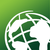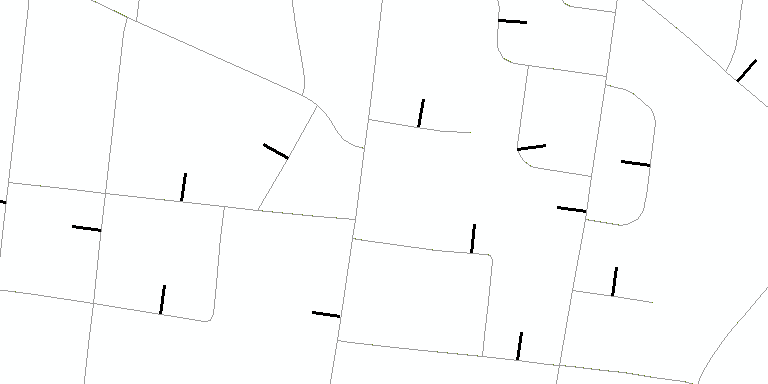# Calculating perpendicular line only works for shapefiles not for feature class

2846
3
02-13-2018 03:38 AMby
New Contributor

In ArcGIS I'm trying to use this field calculator expression on field Shape to generate a perpendicular line, of 0.01m length, at the midpoint of each existing line feature:

``CALCULATE FIELD (data management):Input table: Clip_point_bufferField name: ShapeExpression: RotateExtend( !Shape!, 0.01)Expression type: PythonCode Block:  Pre logic script code:def RotateExtend(plyP,sLength):l=plyP.length ptX=plyP.positionAlongLine (l/2).firstPoint ptX0=plyP.firstPoint ptX1=plyP.lastPoint dX=float(ptX1.X)-float(ptX0.X)dY=float(ptX1.Y)-float(ptX0.Y)lenV=math.sqrt(dX*dX+dY*dY)sX=-dY*sLength/lenV;sY=dX*sLength/lenV leftP=arcpy.Point(ptX.X+sX,ptX.Y+sY)rightP=arcpy.Point(ptX.X-sX, ptX.Y-sY)array = arcpy.Array([leftP,rightP])section=arcpy.Polyline(array)return section Shape:RotateExtend( !Shape!, 0.01)``

It works if I do it with a shapefile, but it doesn't work if I try to do it in this same shapefile imported as a feature class in a database (.gbd). The database has a tolerance 0.001 and the default resolution (0.0001). Why the process does not work when the feature is in a database?

I've read that it might have something to do with the properties of "Shape" type of field, because it's locked when in a database. If that's the error, how could I obtain the same results working with a feature class in a database?

Tags (3)
1 Solution

Accepted SolutionsbyEsri Esteemed Contributor

Below an example of what I mean:

``````def main():
import arcpy

# input and output featureclass
fc_in = r'C:\GeoNet\Streets\GeoNet Street Sample.gdb\LebStreetSample_MP2SP'
fc_out = r'C:\GeoNet\Streets\GeoNet Street Sample.gdb\test_perp_v08'
line_length = 100.0  # lenth of output perpendicular line
tolerance = 1.0  # distance from mid point to determine angle

feats = []
with arcpy.da.SearchCursor(fc_in, ('SHAPE@')) as curs:
for row in curs:
polyline_in = row
polyline_out= GetPerpendicularLine(polyline_in, tolerance, line_length)
feats.append(polyline_out)

arcpy.CopyFeatures_management(feats, fc_out)

def GetPerpendicularLine(polyline, tolerance, length):
pntg1 = polyline.positionAlongLine(polyline.length/2.0 - tolerance, False)
pntg2 = polyline.positionAlongLine(polyline.length/2.0 + tolerance, False)
angle = getAngle(pntg1, pntg2)
angle_perp = angle + 90
pntg_from = polyline.positionAlongLine(0.5, True)
pntg_to = pntg_from.pointFromAngleAndDistance(angle_perp, length, 'PLANAR')
polyline_new = arcpy.Polyline(arcpy.Array([pntg_from.firstPoint, pntg_to.firstPoint]), polyline.spatialReference)
return polyline_new

def getAngle(pntg1, pntg2):
'''determine angle of line based on start and end points geometries'''
return pntg1.angleAndDistanceTo(pntg2, method='PLANAR')

if __name__ == '__main__':
main()‍‍‍‍‍‍‍‍‍‍‍‍‍‍‍‍‍‍‍‍‍‍‍‍‍‍‍‍‍‍‍‍‍‍‍‍``````

Which generates the perpendicular lines and the mid point of the polyline, with the specified length.3 RepliesbyMVP Legendary Contributor

Adri(ana), can you format your code Code Formatting... the Basics so others can try it.

What happens when you change the value to 1.0 or something?

Are you getting error messages? or warnings? or is nothing happening at all?byEsri Esteemed Contributor

You really want to overwrite the geometry using the field calculator. I really hope you are doing this on a back-up of your featureclass... It would be better to run a simple script that creates a new output featureclass with the perpendicular lines.byEsri Esteemed Contributor

Below an example of what I mean:

``````def main():
import arcpy

# input and output featureclass
fc_in = r'C:\GeoNet\Streets\GeoNet Street Sample.gdb\LebStreetSample_MP2SP'
fc_out = r'C:\GeoNet\Streets\GeoNet Street Sample.gdb\test_perp_v08'
line_length = 100.0  # lenth of output perpendicular line
tolerance = 1.0  # distance from mid point to determine angle

feats = []
with arcpy.da.SearchCursor(fc_in, ('SHAPE@')) as curs:
for row in curs:
polyline_in = row
polyline_out= GetPerpendicularLine(polyline_in, tolerance, line_length)
feats.append(polyline_out)

arcpy.CopyFeatures_management(feats, fc_out)

def GetPerpendicularLine(polyline, tolerance, length):
pntg1 = polyline.positionAlongLine(polyline.length/2.0 - tolerance, False)
pntg2 = polyline.positionAlongLine(polyline.length/2.0 + tolerance, False)
angle = getAngle(pntg1, pntg2)
angle_perp = angle + 90
pntg_from = polyline.positionAlongLine(0.5, True)
pntg_to = pntg_from.pointFromAngleAndDistance(angle_perp, length, 'PLANAR')
polyline_new = arcpy.Polyline(arcpy.Array([pntg_from.firstPoint, pntg_to.firstPoint]), polyline.spatialReference)
return polyline_new

def getAngle(pntg1, pntg2):
'''determine angle of line based on start and end points geometries'''
return pntg1.angleAndDistanceTo(pntg2, method='PLANAR')

if __name__ == '__main__':
main()‍‍‍‍‍‍‍‍‍‍‍‍‍‍‍‍‍‍‍‍‍‍‍‍‍‍‍‍‍‍‍‍‍‍‍‍``````

Which generates the perpendicular lines and the mid point of the polyline, with the specified length.